# Operators in C programming

When we code a program, our main goal is to perform some operations on various variables. These operations may be arithmetic or logical operations. In order to perform these operations, we need different operators. These operators are the symbols which informs the compiler about the operation / action to be performed on the variables. There are different types of operations performed in C language. They are:

## Arithmetic Operator

Arithmetic operators are the operators that are used to perform arithmetic operations like addition, subtraction, multiplication, division and modulus (finds the remainder of division).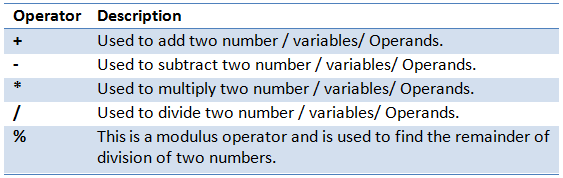int intVar1, intVar2, intResult;
intResult = intVar1 + intVar2;
intResult = intVar1 – intVar2;
intResult = intVar1 * intVar2;
intResult = intVar1 / intVar2;
intResult = intVar1 %intVar2;

## Bitwise Operator

This operator is used to perform the operation on the bit values of the data in the variable.  There are different types of bitwise operators.

### AND (&)

this is the AND operation performed on the bits of the variable. Suppose we have two variables var1 and var2. Then bitwise AND operation on these two variables as shown in below table. It gives result as 1, only if both has bit 1, else the result is 0.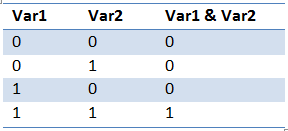Hence if the variables have some non-zero numbers, then it will be first converted into bits, then AND operation is performed on it.

For example, suppose var1 = 20 and var2 = 60, then bitwise AND on these variables is :
var1 =      20 = 0001 0100
var2 =      60 = 0011 1100
var1 & var2= 0001 0100 = 24

### OR(|)

This operator gives result as 1 when at least one of the bits is 1 in the variables. It is denoted by ‘|’.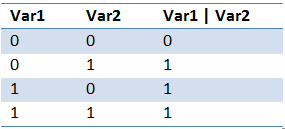var1 =      20 = 0001 0100
var2 =      60 = 0011 1100
var1 | var2= 0011 1100 =60

### XOR (^)

It results in 1 if bits in both the variables are different and if they are same it results in 0. It is denoted by ‘^’.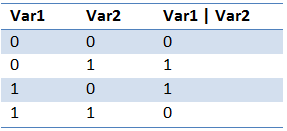var1 =      20 = 0001 0100
var2 =      60 = 0011 1100
var1 ^var2= 0010 1000 =40

### NOT (~)

This operator negates the bit present in each position. That means, it converts 0 to 1and 1 to 0.

Hence ~var1 = ~(0001 0100) = 1110 1011 = 235

### Left Shift Operator (<<

This operator is used to shift the number of bits to the left. The number of bits to be shifted are added on RHS of the operator. As the bits shift to left, 0s will be added to the right.

For example, consider var1 =  20 = 0001 0100
Now var1 << 2 = 0001 0100 << 2 = 0101 0000

### Right Shift Operator (>>)

This is opposite to left shift operator. Here the bits are shifted to right depending on the number of bits specified on the right hand side of the operator. As the bits shift to the right, 0s are added to the left.

For example, consider var1 =  20 = 0001 0100
Now var1 >> 2 = 0001 0100 >> 2 = 0000 0101

## Assignment Operator

Assignment operator is used to assign the result of some operation or expression. One of the simplest and common assignment operator is ‘=’ (equal to). But C allows combining arithmetic operators or bitwise operators with assignment operator to get the results in simple form.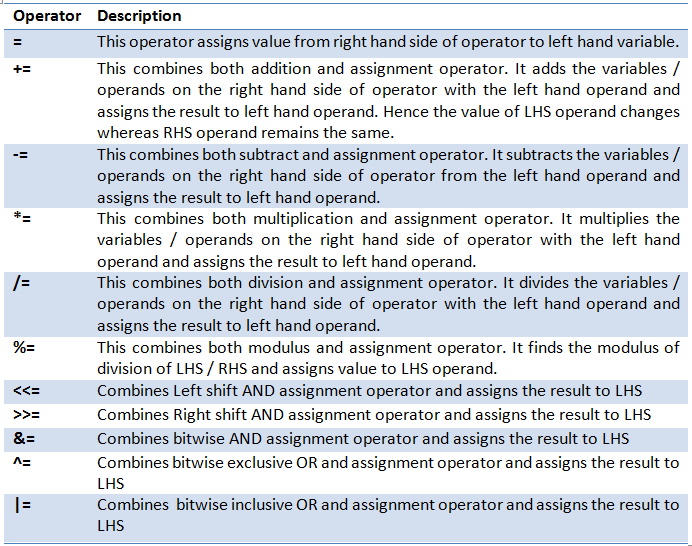int intVar1 = 100, intVar2 = 10;
intVar1 += intVar2; // Result is 110
intVar1 -= intVar2; // Result is 90
intVar1 *= intVar2; // Result is 1000
intVar1 /= intVar2; // Result is 10
intVar1 %= intVar2; // Result is 0

## Increment and Decrement Operator

Whenever we add 1 or subtract 1 from any number or variable, we use arithmetic operator ‘+’ or ‘-‘ respectively. Also, we write full expression as a= b+1 and c = b-1. But C provides another easy mechanism to increment or decrement the numbers by 1 using special operator ‘++’ and ‘—‘ respectively.

For example, let intVar1 = 50. Now if we need to increment the value by 1, then we can write it as below :

intVar1++; // it automatically increases the value of intVar by 1 and assigns the result to intvar1

Similarly, if we have to decrement the value by 1 then, we write:
intVar1–; // it automatically decreases the value of intVar by 1 and assigns the result to intvar1

If we need to increment or decrement by two, then we have to write above expression two times.
Above expression for incrementing and decrementing can be written in below format too.
++intVar1; // it automatically increases the value of intVar by 1 and assigns the result to intvar1

Similarly, if we have to decrement the value by 1 then, we write:
–intVar1; // it automatically decreases the value of intVar by 1 and assigns the result to intvar1

Here result values after both the operations above are same. But only difference is noticed when used in loops or some arithmetic operations. When intVar1++ is used, it first assigns or uses the values present in intVar1 and then increments it by 1 and assigns it to intVar1. When ++intVar1 is used, it first increments the value by 1 and assigns the new value to intVar1, then this new values is used in any calculations/operations. The same holds with decrement operator too.
A simple program below illustrates the same. We can note here that, value displayed in printf statement when intVal++ is used is before incrementing it by 1. When second printf is used, it uses the incremented value, i.e. 26 and then again increments it by 1 and displays result as 27. We can notice the same pattern with decrement operator too.

```#include <stdio.h>

int main(){
int intVal = 25;
printf("\n\nValue of intVal before incrementing is %d \n", intVal);
printf("Value of intVal using increment operator as intVal++ is %d\n", intVal++);
printf("Value of intVal using increment operator as ++intVal is %d\n", ++intVal);

printf("\n\nValue of intVal before decrementing is %d \n", intVal);
printf("Value of intVal using decrement operator as intVal-- is %d\n", intVal--);
printf("Value of intVal using decrement operator as --intVal is %d\n", --intVal);
}```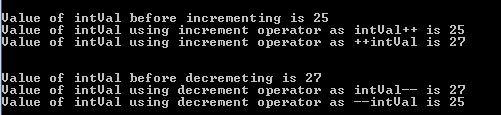## Conditional Operator

This operator is used to check for the conditions. It works same as if condition, but uses the operator like below:

condition ? true_result : false_result;

i.e.; it checks for the condition, if it is correct/ passes then it displays the true_result, else it displays false_result.

chrGrade = (intAvg>80) ? ‘A’ : ‘B’;
*chrNumType = (intVal >= 0) ? “Positive” : “Negative”;

## Relational Operator

These operators are used to check equality, non-equality, less than, less than equal to, greater than, greater than or equal of any two numeric variables. Usually these operators are used while performing the condition checks in if statements and while loops. This is because, the result of these operators is always TRUE or FALSE.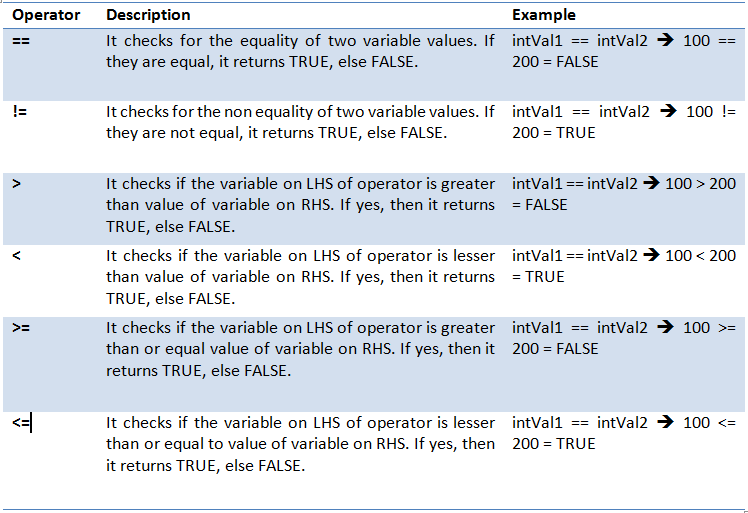```if(intVal1 == intVal2) {
printf("Both the values are same!");
}else{
printf("Both the values are NOT same!");
}```

## Logical Operator

These operators are used along with relational operators. To be more specific, these operators combine the conditions of relational operators by using AND operator (&&), or considers either of relational conditions using OR operator (||) or it negates the relational condition by using NOT operator (!). these are mainly used while checking the conditions in if statements and for / while loops.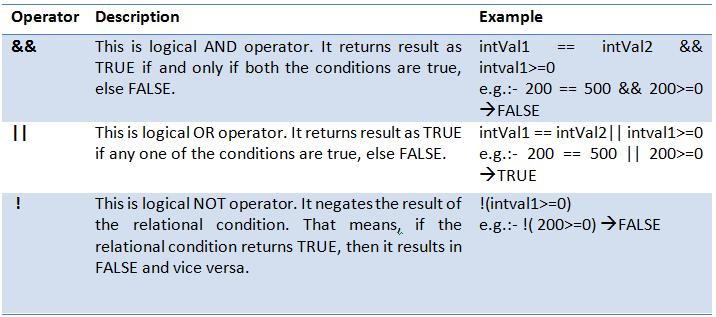Please note here that both bitwise operator and logical operator uses the same logic to evaluate the expression. But bitwise operator is applied on the bits – basic representation of data, whereas logical operators works on statements and expressions. Hence both of these operators are different, even though the logic is same.

## Miscellaneous Operator

Apart from above listed operators, there are few other operators used in C language.

### sizeof ()

this is used to find the size of the variables in C. These variables can be any type of variable from primitive, non-primitive to user-defined type of variable. We can even use it for finding the size of datatypes too. For example,

```printf("Size of integer is %d", sizeof(int)); // displays ‘Size of integer is 4’
printf("Size of chrOption is %d", sizeof(chrOption)); // displays ‘Size of chrOption is 1’
printf("Size of strStudent is %d", sizeof(strStudent)); // displays ‘Size of strStudent is 33’
(structure size)```

When we use sizeof operator to get the size of any type and assign it to any other variable, then we can assign it to a type size_t. This is a type defined in stddef.h header file. In this file it is defined as unsigned int type (it is declared using typedef keyword; hence the type is size_t). But it can be unsigned short int or unsigned long it depending on the system. This operator can be used to get the size of any variable, array, structure, union, pointers etc too.

While using the sizeof operator on arrays, care should be taken to not to use it when array is a function parameter. It will not give the correct result in this case.

This operator returns the address of the variable. It is appended before the variable name to find the address of the variable.

```printf("Address of chrOption is %x", &chrOption); // displays address of chrOption
scanf("%d", &intVal); // input value is entered at the address of intVal```

### Pointer Operator (*)

This operator when appended before variable name, acts as a pointer variable.

```int *intPtr;
float *flArray ;
char *chrName;```
Translate »